# NCERT Exemplar Class 10 Maths Solutions for Chapter 13 - Statistics And Probability

## NCERT Exemplar Solutions Class 10 Maths Chapter 13 – Free PDF Download

NCERT Exemplar Class 10 Maths Chapter 13 Statistics and Probability, provided here for students to prepare for CBSE exams. These problems and solutions are prepared by our subject experts in accordance with CBSE latest syllabus (2021-2022), to help students score better. Students can use the NCERT Exemplar for Class 10 Maths as a productive tool for studying as well as practising sums. The exemplar also contains solved questions relevant to the exercise problems present in the textbook. Moreover, it will allow students to have a thorough revision of the entire chapter and be prepared to face the exams.

Chapter 13 basically has problems based on the different statistical measures like mean, mode, and median. Students will learn about how to solve these problems and also about the concept of cumulative frequency distribution, cumulative frequency curves, and more. Additionally, students will also develop an experimental approach to probability. Students will explore concepts like multiplication rule of probability, the Bayes’ theorem, and independence of events. These Exemplars problems and solutions cover the following topics of Statistics and Probability given below:

• Determining the mean of grouped data by a direct method, assumed mean method, step-deviation method
• Finding the mode of the given data
• To find the median of the grouped data
• Representation of Cumulative Frequency Distribution graphically

## Download PDF of NCERT Exemplar Class 10 Maths Solutions for Chapter 13 Statistics and Probability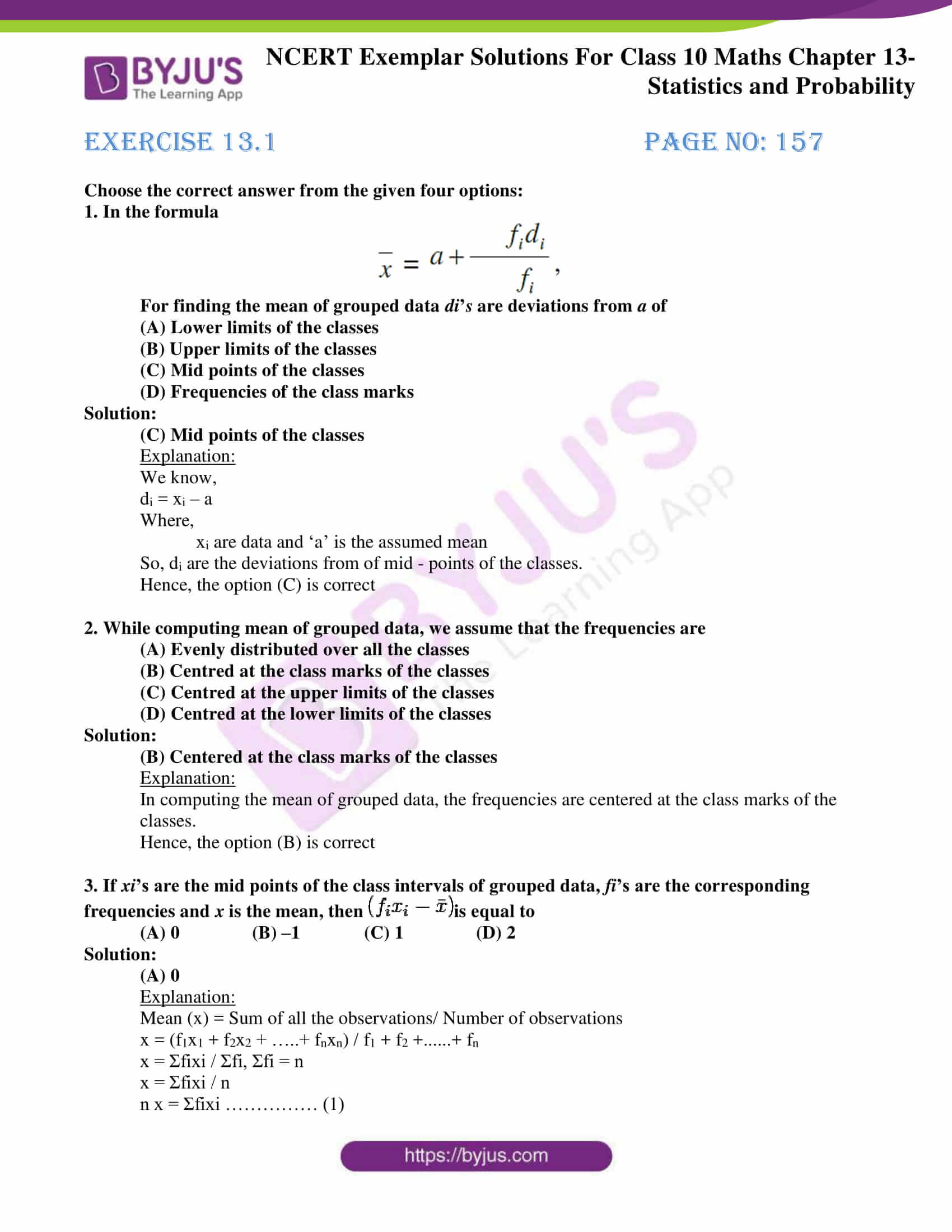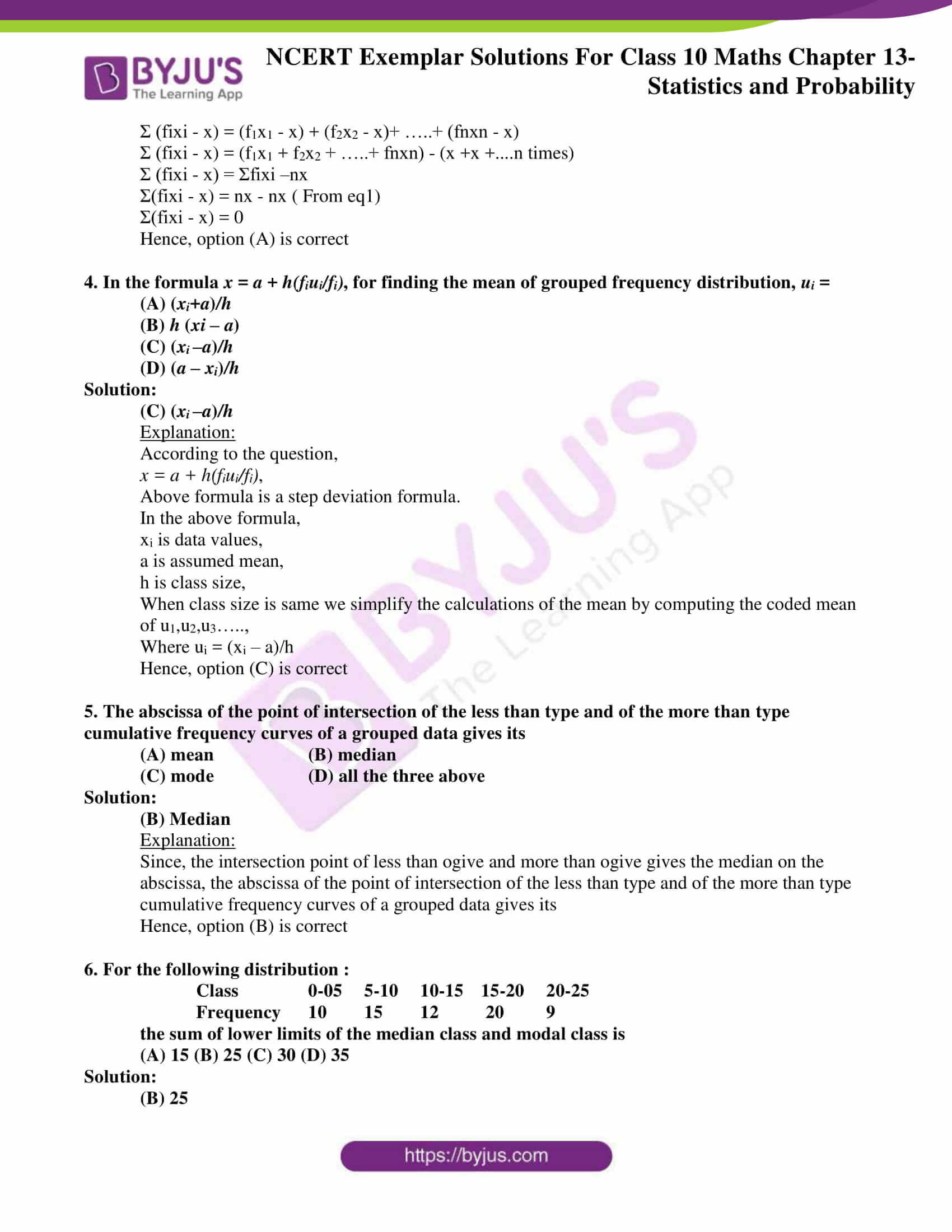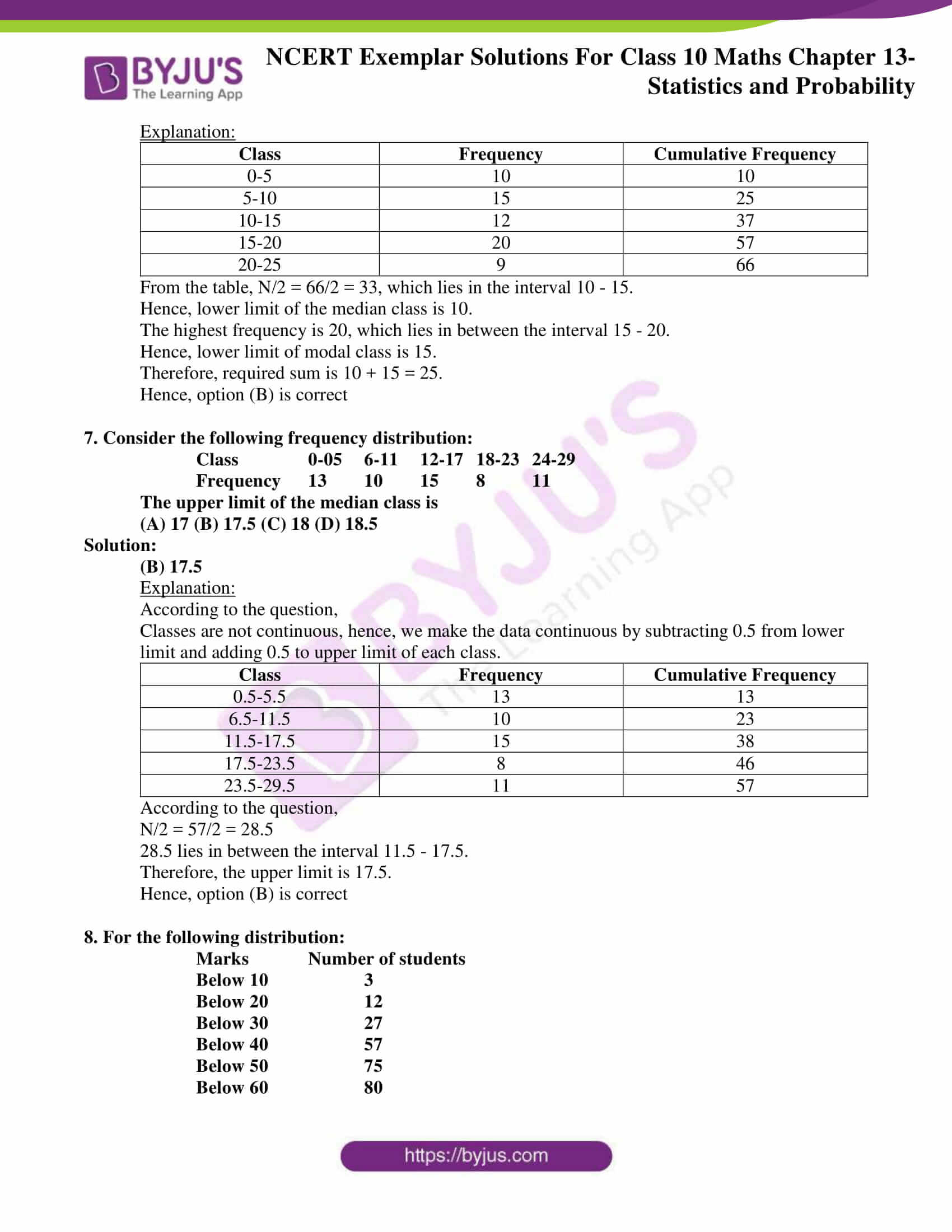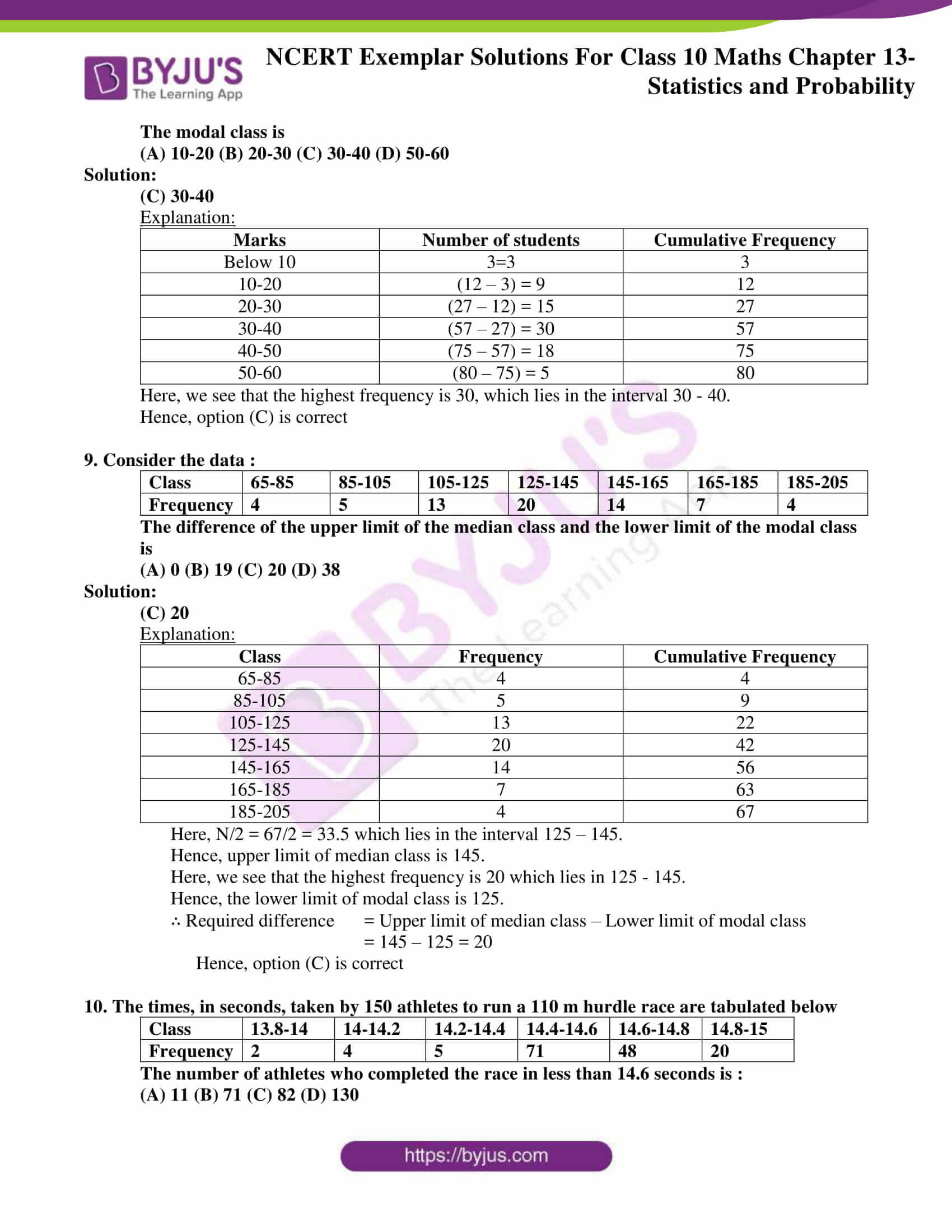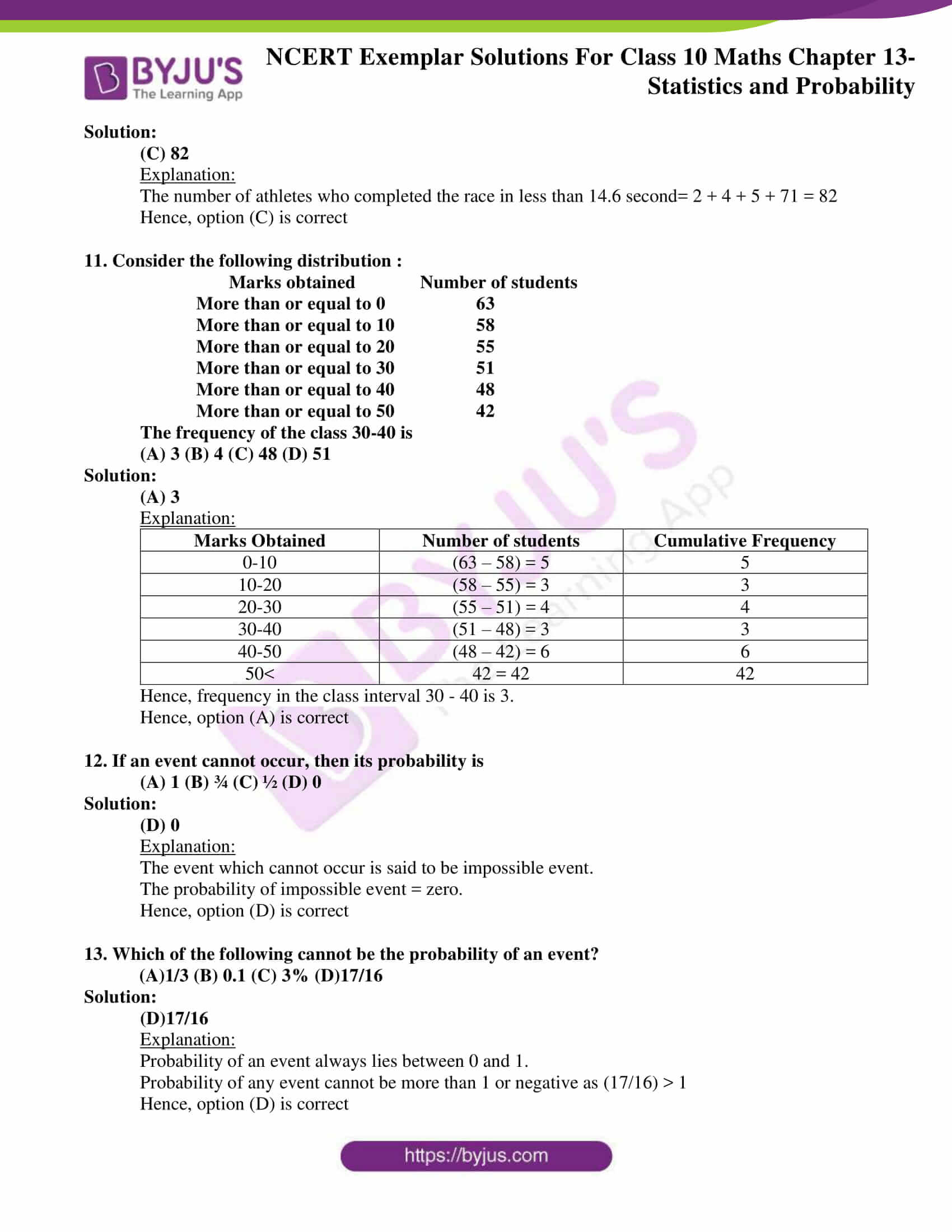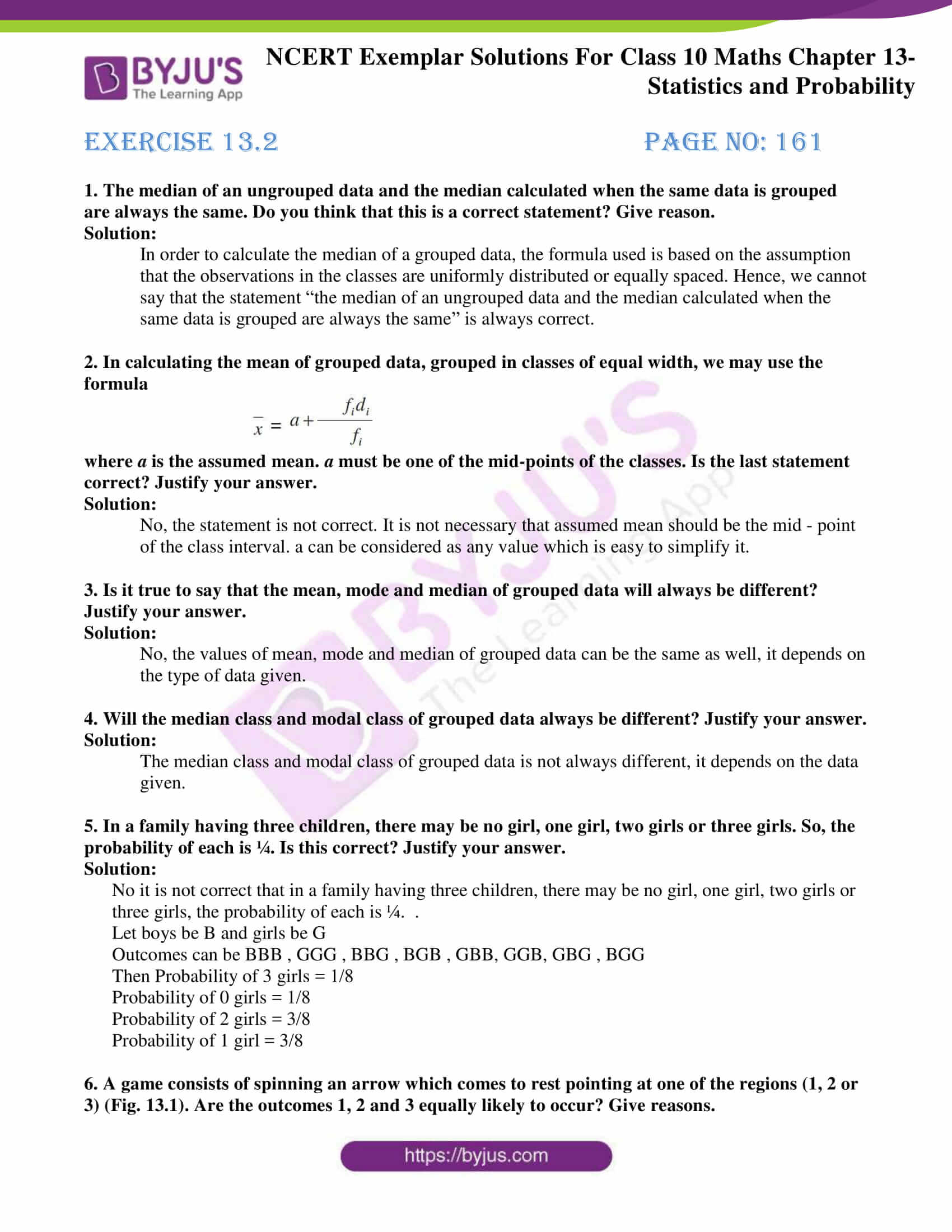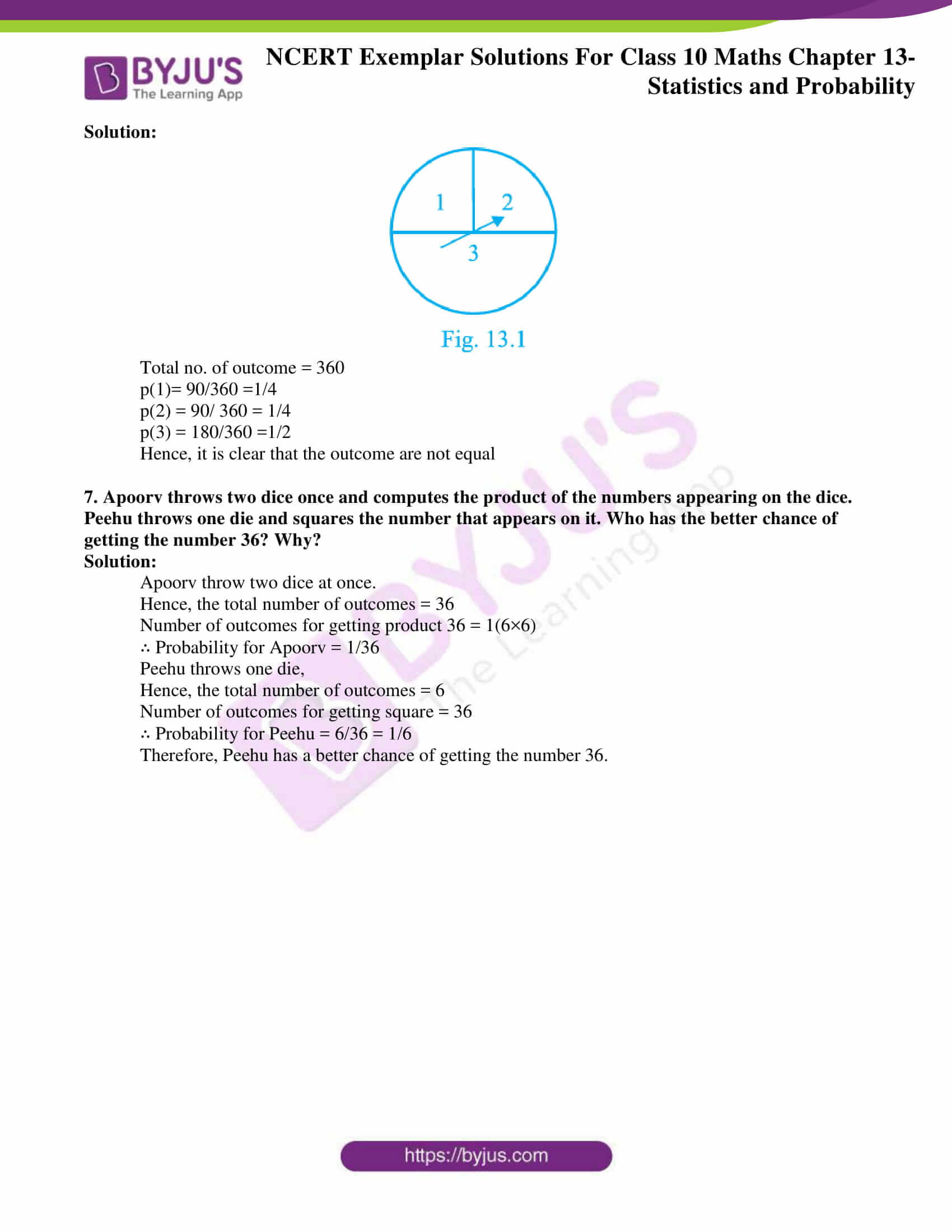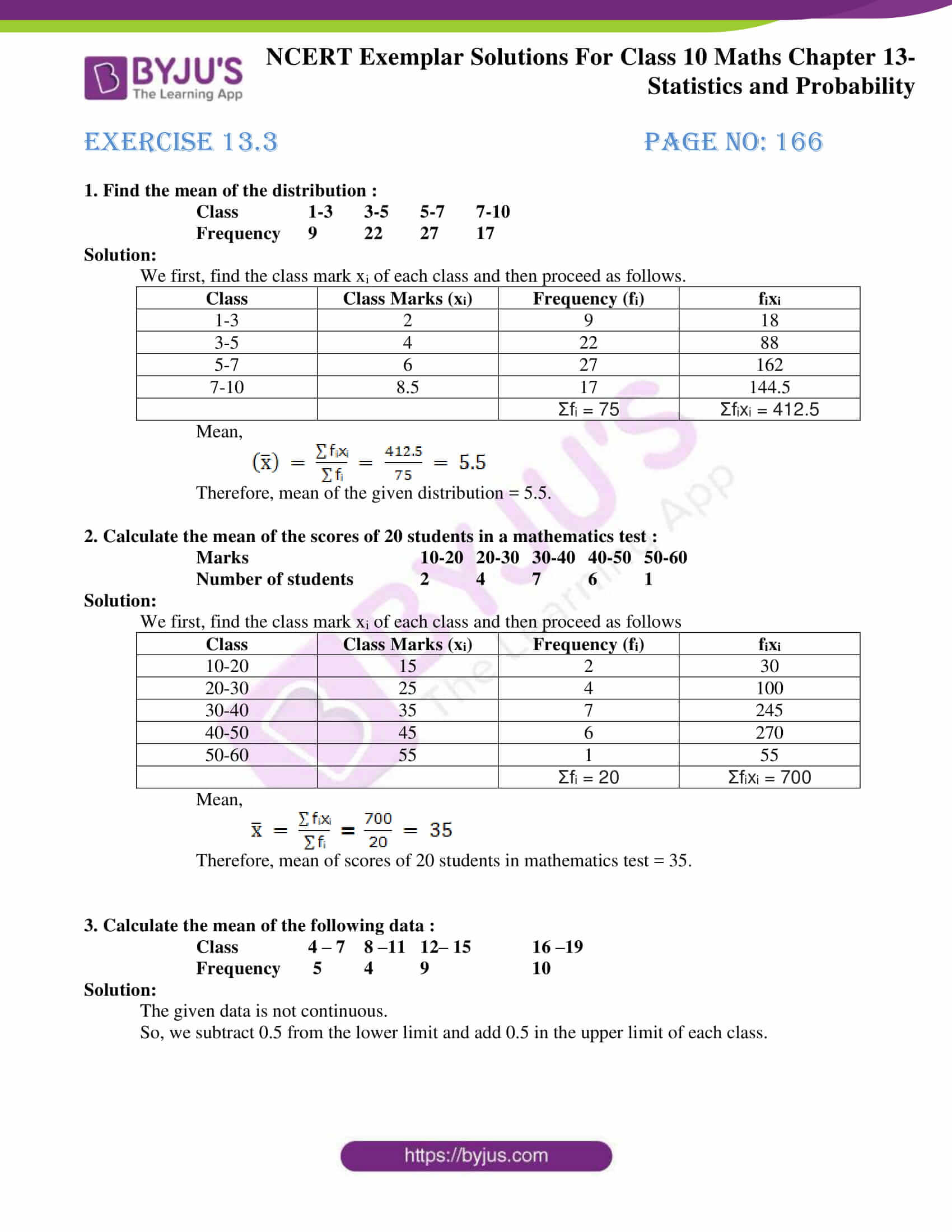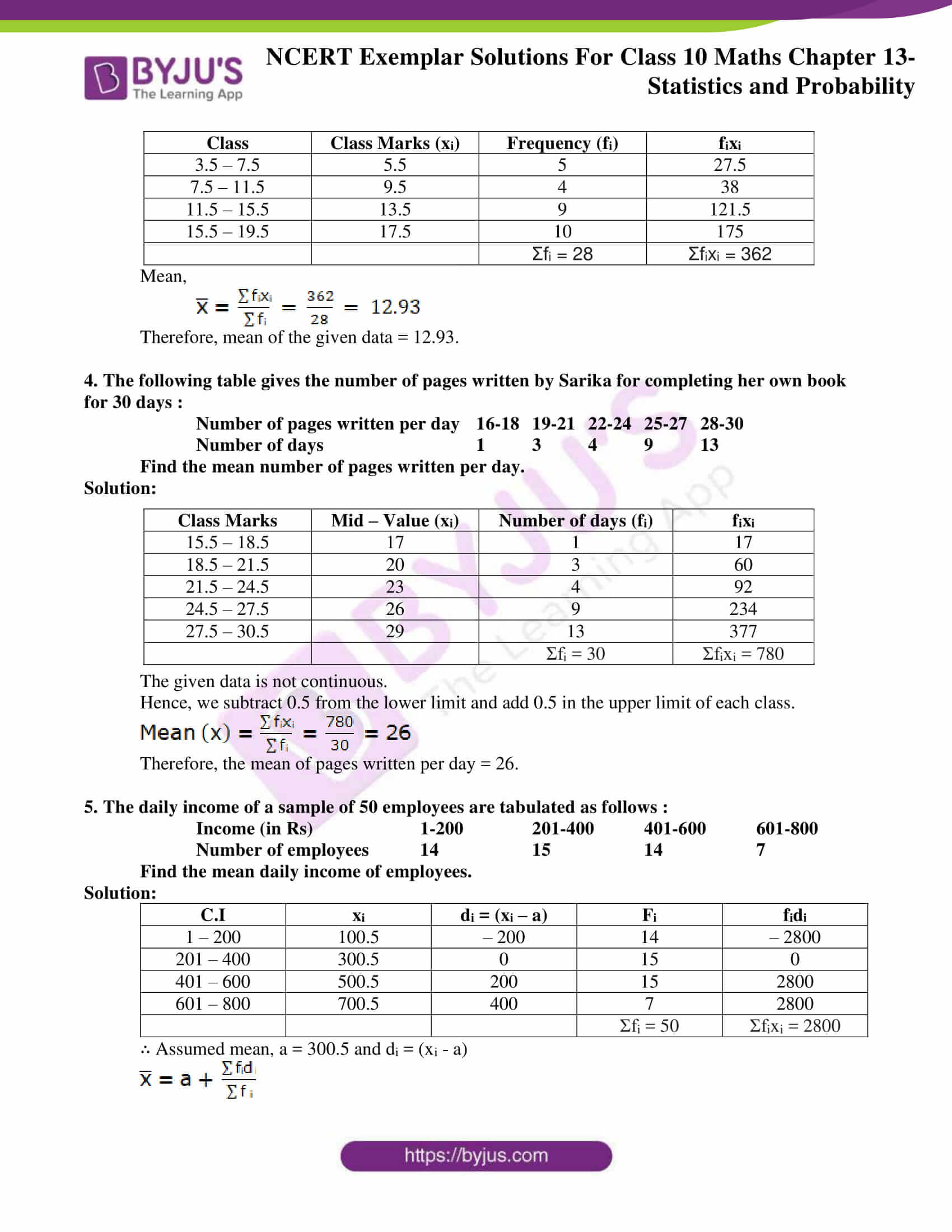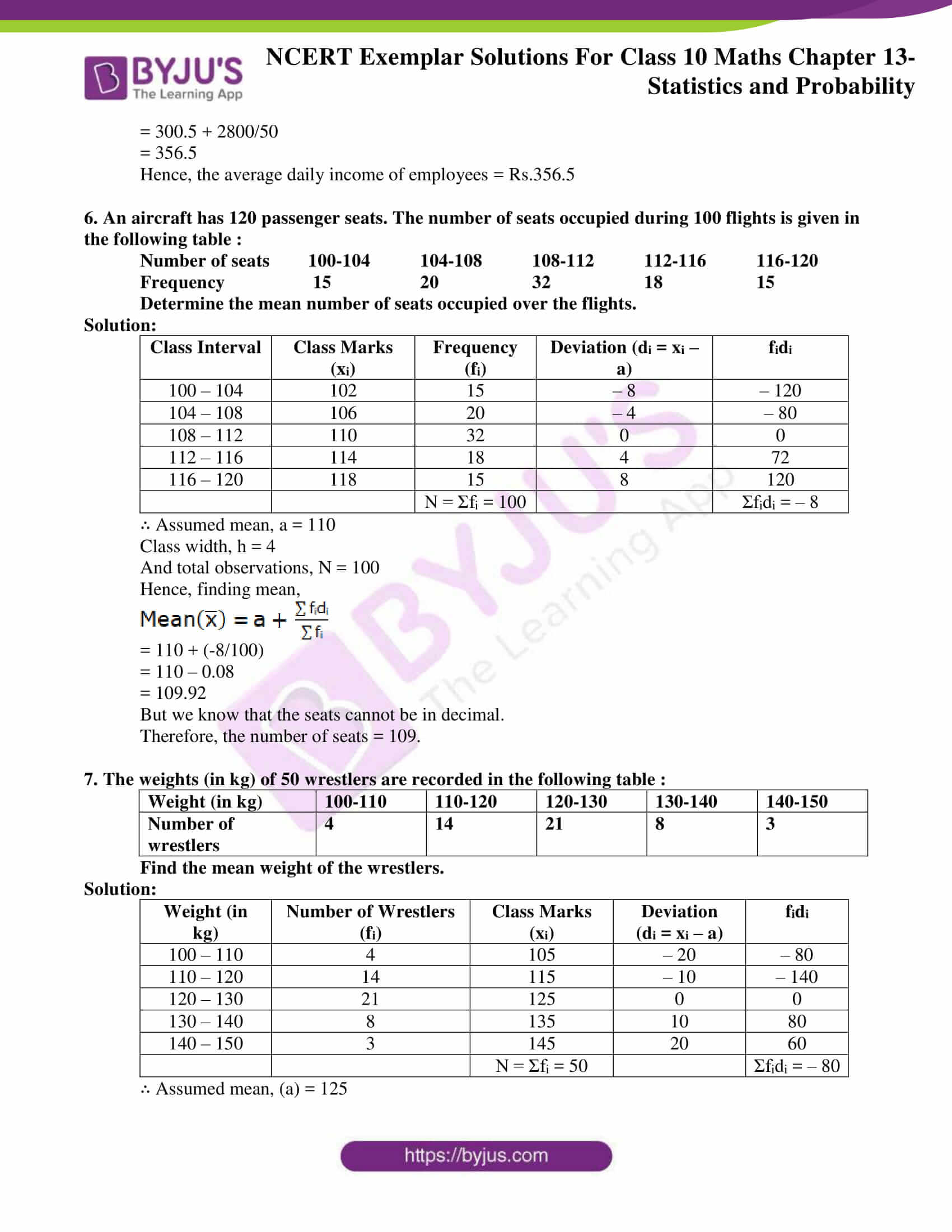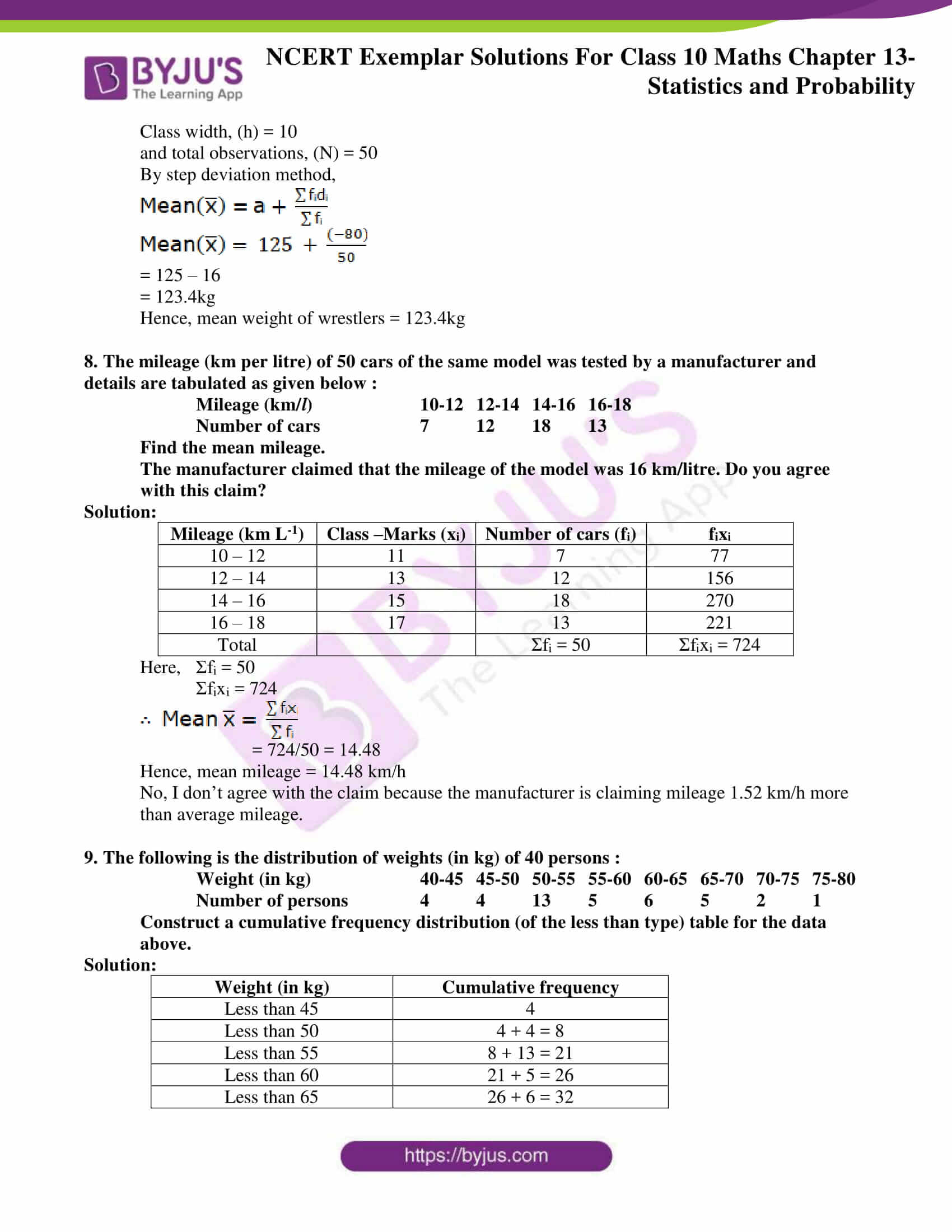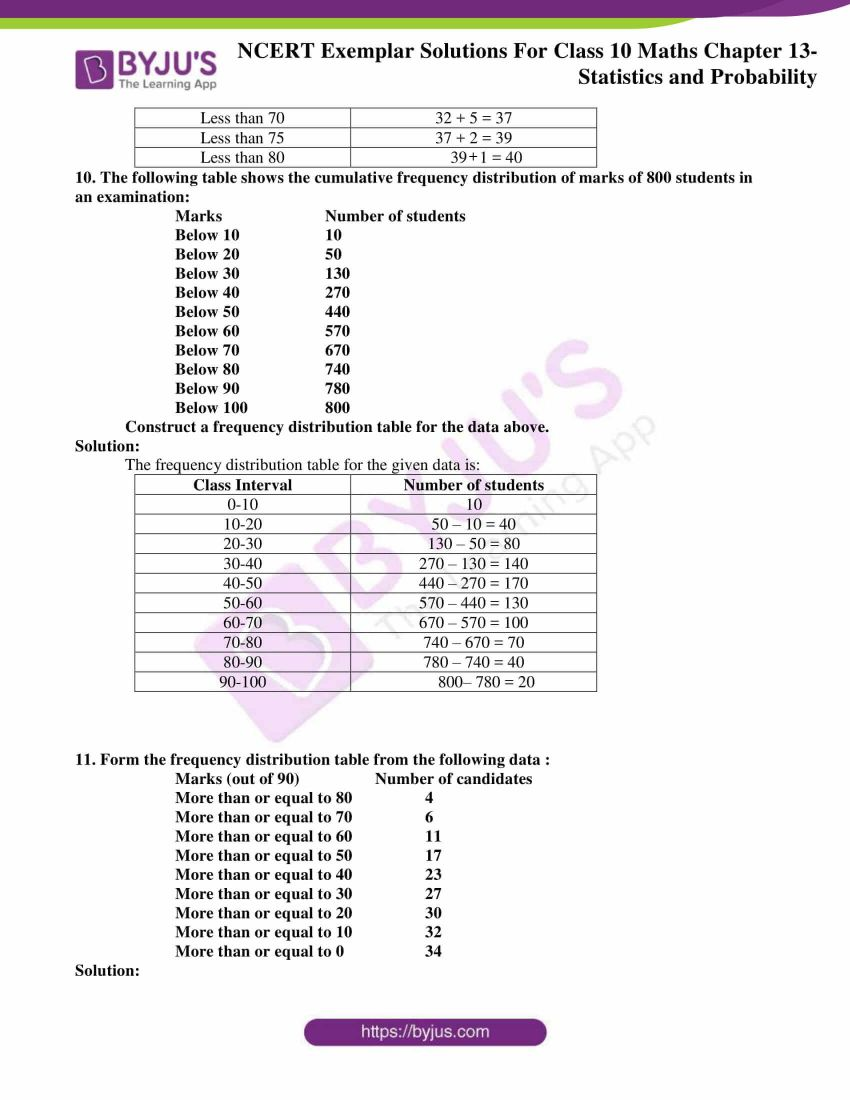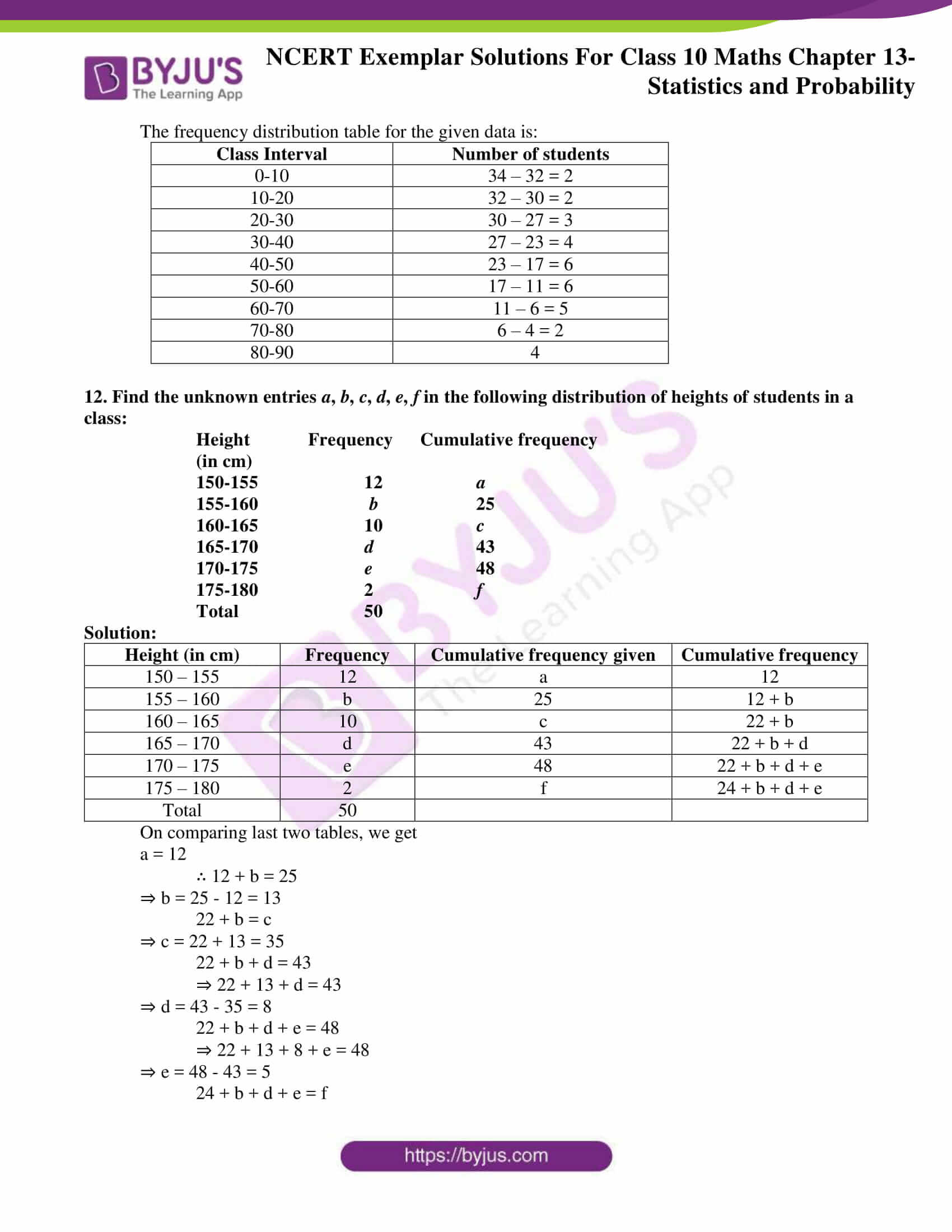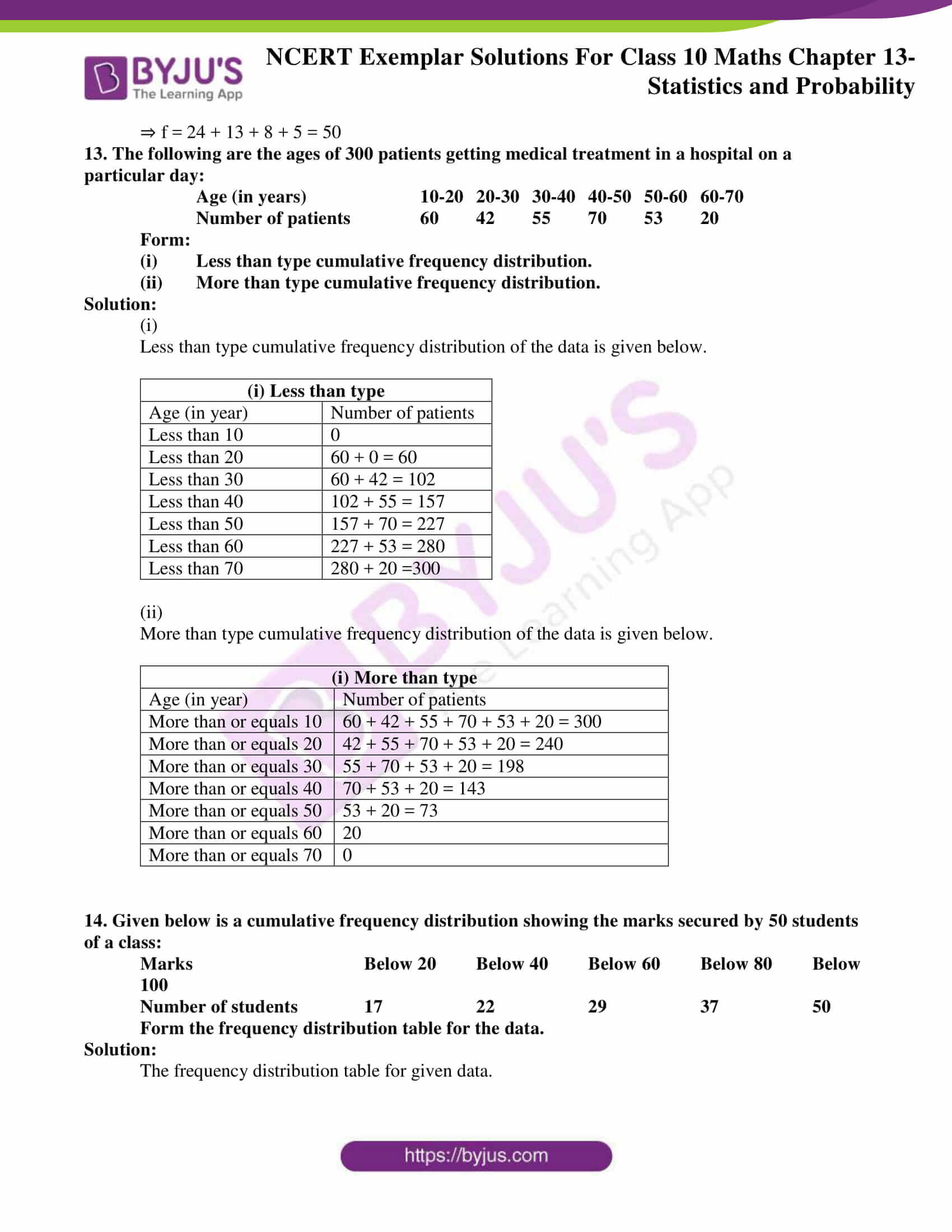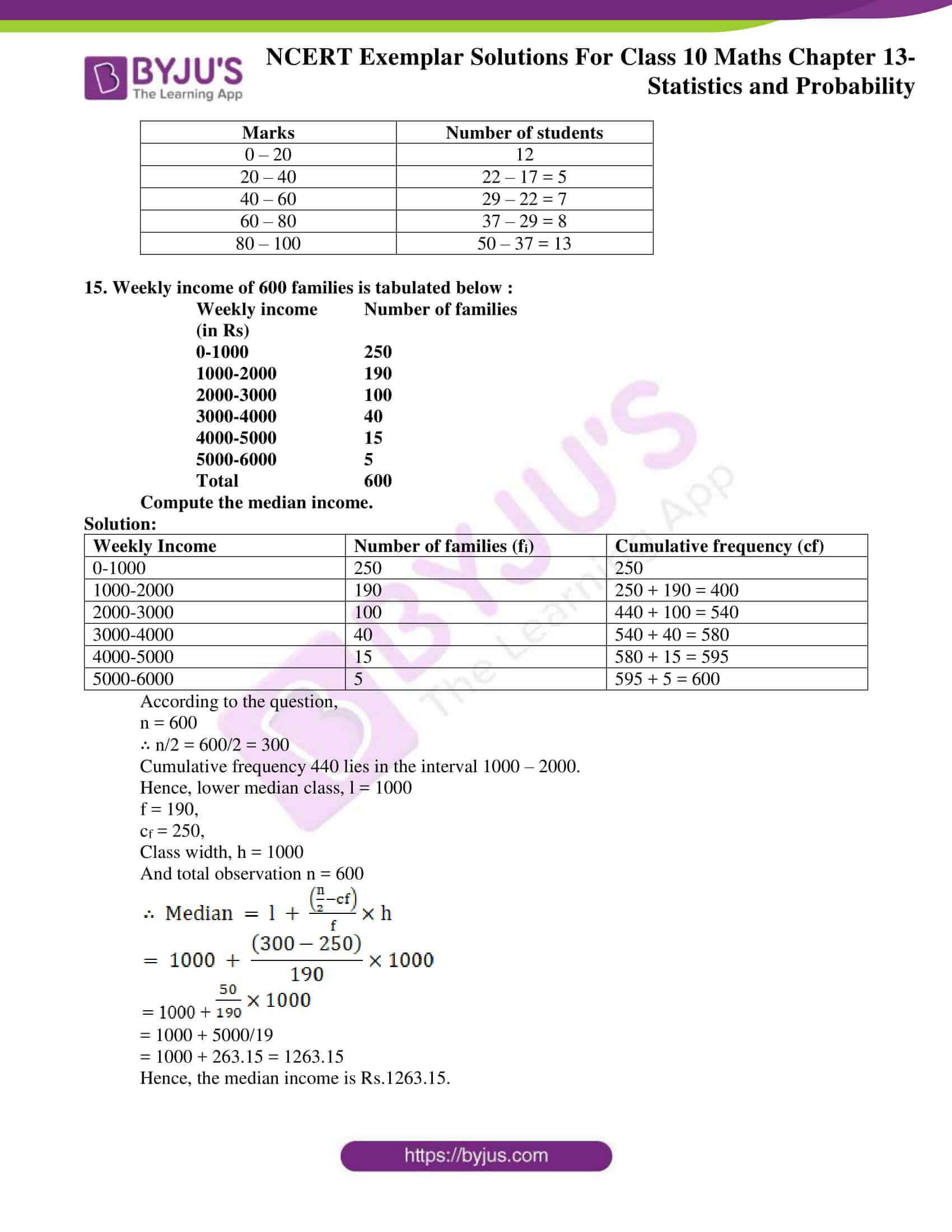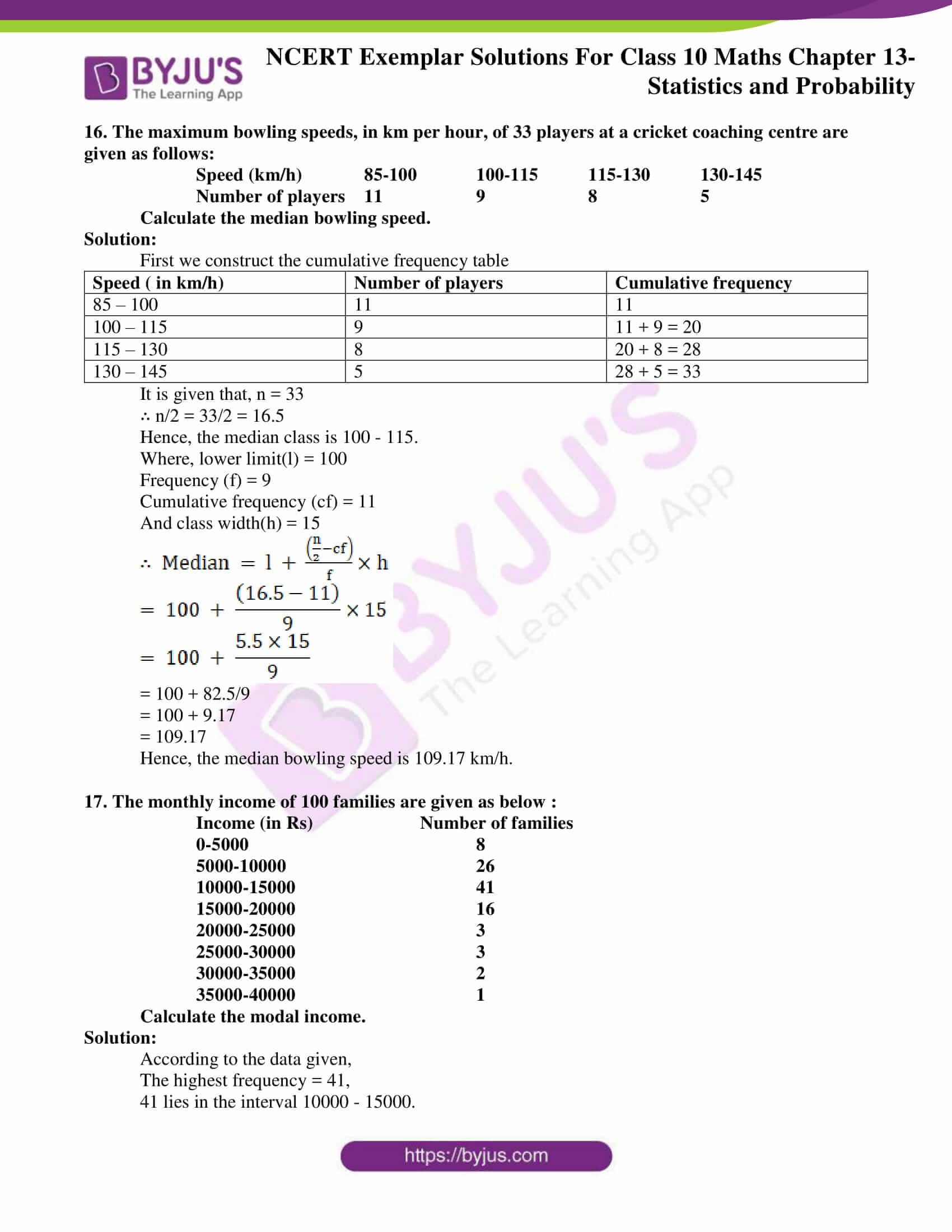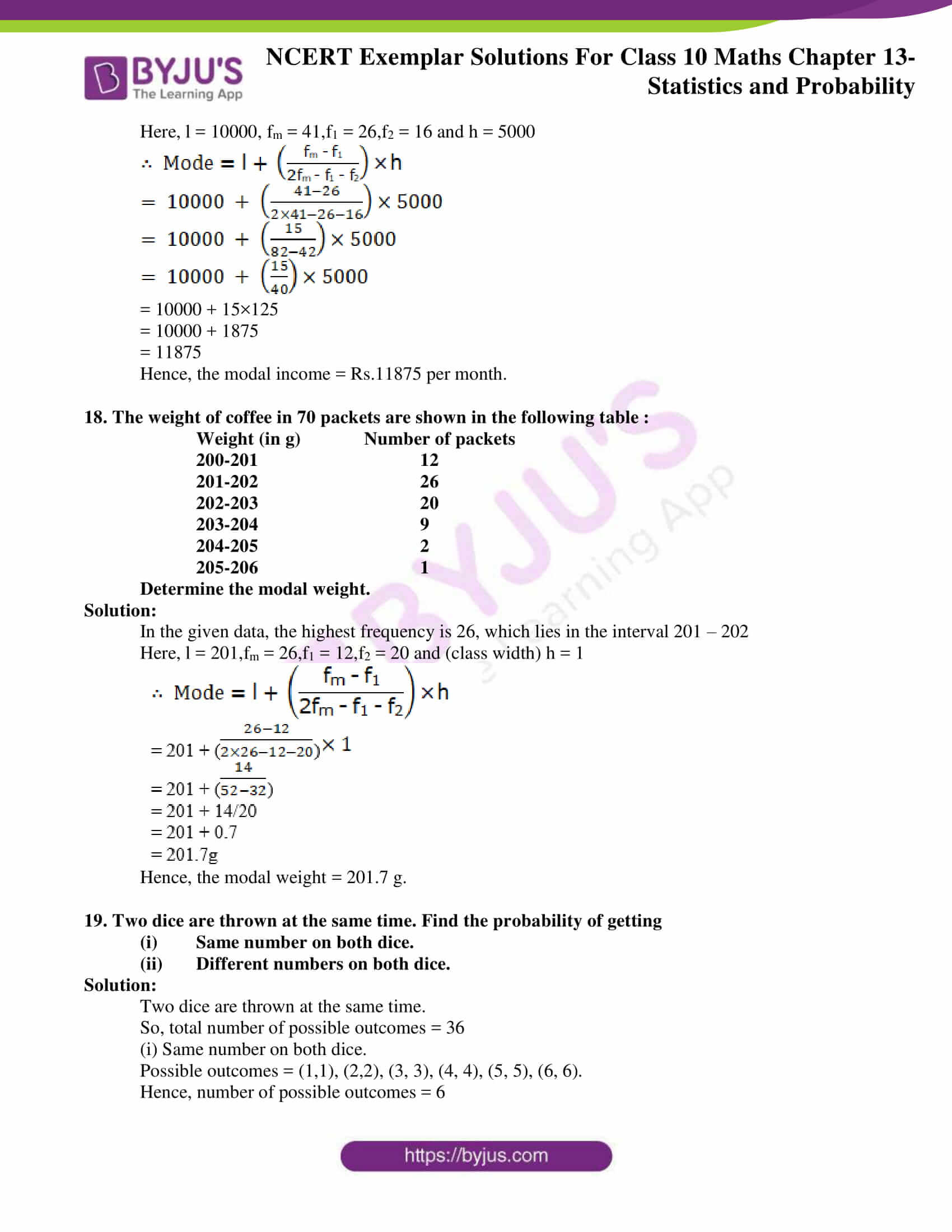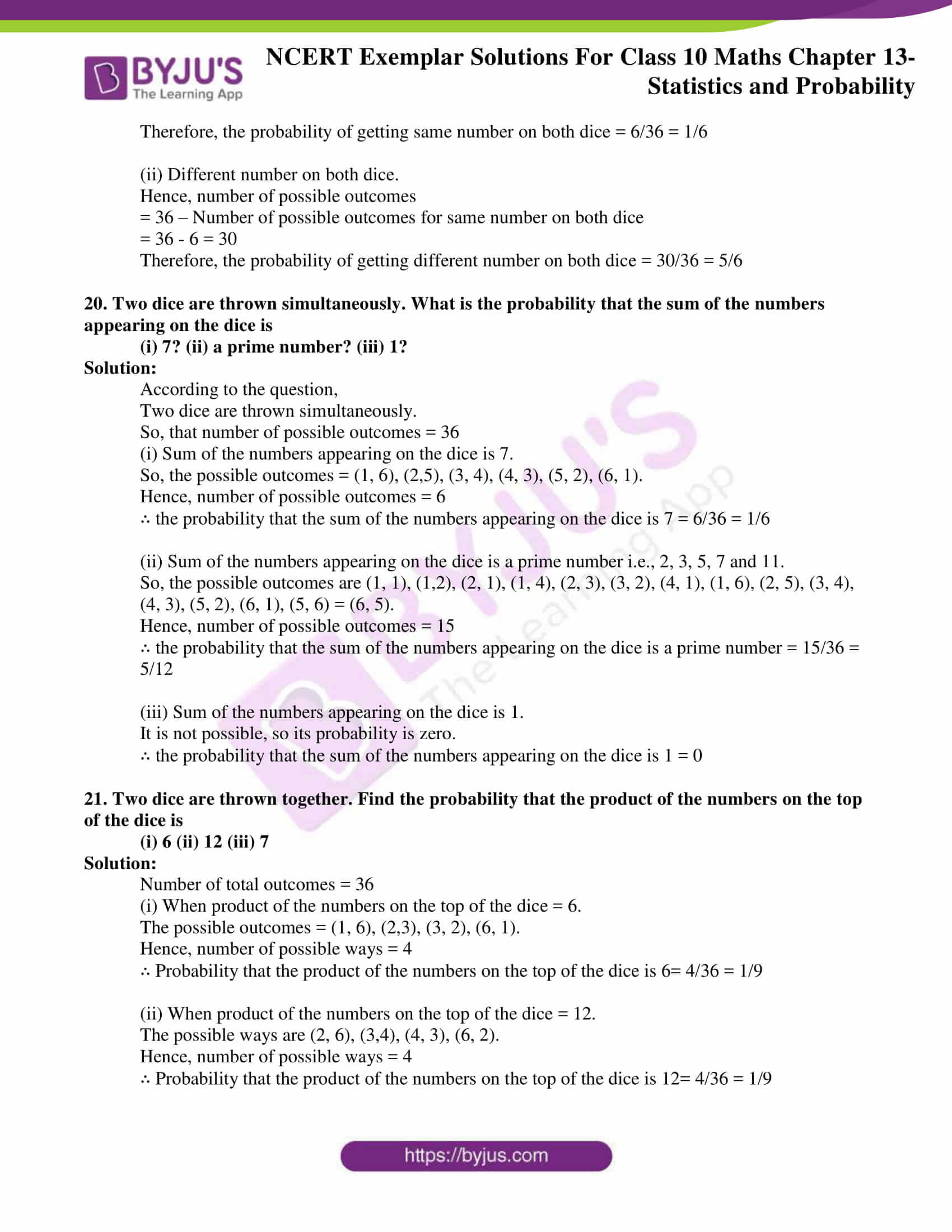## Exercise 13.1 Page No: 157

Choose the correct answer from the given four options:

1. In the formula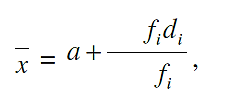For finding the mean of grouped data dis are deviations from a of

(A) Lower limits of the classes

(B) Upper limits of the classes

(C) Mid points of the classes

(D) Frequencies of the class marks

Solution:

(C) Mid points of the classes

Explanation:

We know,

di = xi – a

Where,

xi are data and ‘a’ is the assumed mean

So, di are the deviations from of mid – points of the classes.

Hence, the option (C) is correct

2. While computing mean of grouped data, we assume that the frequencies are

(A) Evenly distributed over all the classes

(B) Centred at the class marks of the classes

(C) Centred at the upper limits of the classes

(D) Centred at the lower limits of the classes

Solution:

(B) Centered at the class marks of the classes

Explanation:

In computing the mean of grouped data, the frequencies are centered at the class marks of the classes.

Hence, the option (B) is correct

3. If xi’s are the mid points of the class intervals of grouped data, fi’s are the corresponding frequencies and x is the mean, then (fixi

$$\begin{array}{l}\overline{x}\end{array}$$
) is equal to

(A)0 (B) –1 (C) 1 (D) 2

Solution:

(A) 0

Explanation:

Mean (x) = Sum of all the observations/ Number of observations

x = (f1x1 + f2x2 + …..+ fnxn) / f1 + f2 +……+ fn

x = Σfixi / Σfi, Σfi = n

x = Σfixi / n

n x = Σfixi …………… (1)

Σ (fixi – x) = (f1x1 – x) + (f2x2 – x)+ …..+ (fnxn – x)

Σ (fixi – x) = (f1x1 + f2x2 + …..+ fnxn) – (x +x +….n times)

Σ (fixi – x) = Σfixi –nx

Σ(fixi – x) = nx – nx ( From eq1)

Σ(fixi – x) = 0

Hence, option (A) is correct

4. In the formula x = a + h(fiui/fi), for finding the mean of grouped frequency distribution, ui =

(A) (xi+a)/h

(B) h (xi a)

(C) (xi –a)/h

(D) (a – xi)/h

Solution:

(C) (xi –a)/h

Explanation:

According to the question,

x = a + h(fiui/fi),

Above formula is a step deviation formula.

In the above formula,

xi is data values,

a is assumed mean,

h is class size,

When class size is same we simplify the calculations of the mean by computing the coded mean of u1,u2,u3…..,

Where ui = (xi – a)/h

Hence, option (C) is correct

5. The abscissa of the point of intersection of the less than type and of the more than type cumulative frequency curves of a grouped data gives its

(A) mean (B) median

(C) mode (D) all the three above

Solution:

(B) Median

Explanation:

Since, the intersection point of less than ogive and more than ogive gives the median on the abscissa, the abscissa of the point of intersection of the less than type and of the more than type cumulative frequency curves of a grouped data gives its

Hence, option (B) is correct

6. For the following distribution :

Class 0-05 5-10 10-15 15-20 20-25

Frequency 10 15 12 20 9

the sum of lower limits of the median class and modal class is

(A)15 (B) 25 (C) 30 (D) 35

Solution:

(B) 25

Explanation:

 Class Frequency Cumulative Frequency 0-5 10 10 5-10 15 25 10-15 12 37 15-20 20 57 20-25 9 66

From the table, N/2 = 66/2 = 33, which lies in the interval 10 – 15.

Hence, lower limit of the median class is 10.

The highest frequency is 20, which lies in between the interval 15 – 20.

Hence, lower limit of modal class is 15.

Therefore, required sum is 10 + 15 = 25.

Hence, option (B) is correct

7. Consider the following frequency distribution:

Class 0-05 6-11 12-17 18-23 24-29

Frequency 13 10 15 8 11

The upper limit of the median class is

(A)17 (B) 17.5 (C) 18 (D) 18.5

Solution:

(B) 17.5

Explanation:

According to the question,

Classes are not continuous, hence, we make the data continuous by subtracting 0.5 from lower limit and adding 0.5 to upper limit of each class.

 Class Frequency Cumulative Frequency 0.5-5.5 13 13 6.5-11.5 10 23 11.5-17.5 15 38 17.5-23.5 8 46 23.5-29.5 11 57

According to the question,

N/2 = 57/2 = 28.5

28.5 lies in between the interval 11.5 – 17.5.

Therefore, the upper limit is 17.5.

Hence, option (B) is correct

8. For the following distribution:

Marks Number of students

Below 10 3

Below 20 12

Below 30 27

Below 40 57

Below 50 75

Below 60 80

The modal class is

(A)10-20 (B) 20-30 (C) 30-40 (D) 50-60

Solution:

(C) 30-40

Explanation:

 Marks Number of students Cumulative Frequency Below 10 3=3 3 10-20 (12 – 3) = 9 12 20-30 (27 – 12) = 15 27 30-40 (57 – 27) = 30 57 40-50 (75 – 57) = 18 75 50-60 (80 – 75) = 5 80

Here, we see that the highest frequency is 30, which lies in the interval 30 – 40.

Hence, option (C) is correct

9. Consider the data :

 Class 65-85 85-105 105-125 125-145 145-165 165-185 185-205 Frequency 4 5 13 20 14 7 4

The difference of the upper limit of the median class and the lower limit of the modal class is

A0 (B) 19 (C) 20 (D) 38

Solution:

(C) 20

Explanation:

 Class Frequency Cumulative Frequency 65-85 4 4 85-105 5 9 105-125 13 22 125-145 20 42 145-165 14 56 165-185 7 63 185-205 4 67

Here, N/2 = 67/2 = 33.5 which lies in the interval 125 – 145.

Hence, upper limit of median class is 145.

Here, we see that the highest frequency is 20 which lies in 125 – 145.

Hence, the lower limit of modal class is 125.

∴ Required difference = Upper limit of median class – Lower limit of modal class

= 145 – 125 = 20

Hence, option (C) is correct

10. The times, in seconds, taken by 150 athletes to run a 110 m hurdle race are tabulated below

 Class 13.8-14 14-14.2 14.2-14.4 14.4-14.6 14.6-14.8 14.8-15 Frequency 2 4 5 71 48 20

The number of athletes who completed the race in less than 14.6 seconds is :

A11 (B) 71 (C) 82 (D) 130

Solution:

(C) 82

Explanation:

The number of athletes who completed the race in less than 14.6 second= 2 + 4 + 5 + 71 = 82

Hence, option (C) is correct

11. Consider the following distribution :

Marks obtained Number of students

More than or equal to 0 63

More than or equal to 10 58

More than or equal to 20 55

More than or equal to 30 51

More than or equal to 40 48

More than or equal to 50 42

The frequency of the class 30-40 is

(A) 3 (B) 4 (C) 48 (D) 51

Solution:

(A) 3

Explanation:

 Marks Obtained Number of students Cumulative Frequency 0-10 (63 – 58) = 5 5 10-20 (58 – 55) = 3 3 20-30 (55 – 51) = 4 4 30-40 (51 – 48) = 3 3 40-50 (48 – 42) = 6 6 50< 42 = 42 42

Hence, frequency in the class interval 30 – 40 is 3.

Hence, option (A) is correct

12. If an event cannot occur, then its probability is

(A)1 (B) ¾ (C) ½ (D) 0

Solution:

(D) 0

Explanation:

The event which cannot occur is said to be impossible event.

The probability of impossible event = zero.

Hence, option (D) is correct

13. Which of the following cannot be the probability of an event?

(A)1/3 (B) 0.1 (C) 3% (D)17/16

Solution:

(D)17/16

Explanation:

Probability of an event always lies between 0 and 1.

Probability of any event cannot be more than 1 or negative as (17/16) > 1

Hence, option (D) is correct

## Exercise 13.2 Page No: 161

1. The median of an ungrouped data and the median calculated when the same data is grouped are always the same. Do you think that this is a correct statement? Give reason.

Solution:

In order to calculate the median of a grouped data, the formula used is based on the assumption that the observations in the classes are uniformly distributed or equally spaced. Hence, we cannot say that the statement “the median of an ungrouped data and the median calculated when the same data is grouped are always the same” is always correct.

2. In calculating the mean of grouped data, grouped in classes of equal width, we may use the formula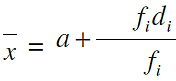where a is the assumed mean. a must be one of the mid-points of the classes. Is the last statement correct? Justify your answer.

Solution:

No, the statement is not correct. It is not necessary that assumed mean should be the mid – point of the class interval. a can be considered as any value which is easy to simplify it.

3. Is it true to say that the mean, mode and median of grouped data will always be different? Justify your answer.

Solution:

No, the values of mean, mode and median of grouped data can be the same as well, it depends on the type of data given.

4. Will the median class and modal class of grouped data always be different? Justify your answer.

Solution:

The median class and modal class of grouped data is not always different, it depends on the data given.

5. In a family having three children, there may be no girl, one girl, two girls or three girls. So, the probability of each is ¼. Is this correct? Justify your answer.

Solution:

No it is not correct that in a family having three children, there may be no girl, one girl, two girls or three girls, the probability of each is ¼. .

Let boys be B and girls be G

Outcomes can be BBB , GGG , BBG , BGB , GBB, GGB, GBG , BGG

Then Probability of 3 girls = 1/8

Probability of 0 girls = 1/8

Probability of 2 girls = 3/8

Probability of 1 girl = 3/8

6. A game consists of spinning an arrow which comes to rest pointing at one of the regions (1, 2 or 3) (Fig. 13.1). Are the outcomes 1, 2 and 3 equally likely to occur? Give reasons.

Solution: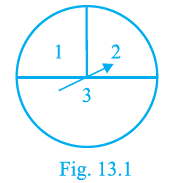Total no. of outcome = 360

p(1)= 90/360 =1/4

p(2) = 90/ 360 = 1/4

p(3) = 180/360 =1/2

Hence, it is clear that the outcome are not equal

7. Apoorv throws two dice once and computes the product of the numbers appearing on the dice. Peehu throws one die and squares the number that appears on it. Who has the better chance of getting the number 36? Why?

Solution:

Apoorv throw two dice at once.

Hence, the total number of outcomes = 36

Number of outcomes for getting product 36 = 1(6×6)

∴ Probability for Apoorv = 1/36

Peehu throws one die,

Hence, the total number of outcomes = 6

Number of outcomes for getting square = 36

∴ Probability for Peehu = 6/36 = 1/6

Therefore, Peehu has a better chance of getting the number 36.

## Exercise 13.3 Page No: 166

1. Find the mean of the distribution :

Class 1-3 3-5 5-7 7-10

Frequency 9 22 27 17

Solution:

We first, find the class mark xi of each class and then proceed as follows.

 Class Class Marks (xi) Frequency (fi) fixi 1-3 2 9 18 3-5 4 22 88 5-7 6 27 162 7-10 8.5 17 144.5 Σfi = 75 Σfixi = 412.5

Mean,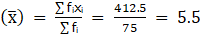Therefore, mean of the given distribution = 5.5.

2. Calculate the mean of the scores of 20 students in a mathematics test :

Marks 10-20 20-30 30-40 40-50 50-60

Number of students 2 4 7 6 1

Solution:

We first, find the class mark xi of each class and then proceed as follows

 Class Class Marks (xi) Frequency (fi) fixi 10-20 15 2 30 20-30 25 4 100 30-40 35 7 245 40-50 45 6 270 50-60 55 1 55 Σfi = 20 Σfixi = 700

Mean,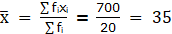Therefore, mean of scores of 20 students in mathematics test = 35.

3. Calculate the mean of the following data :

Class 4 – 7 8 –11 12– 15 16 –19

Frequency 5 4 9 10

Solution:

The given data is not continuous.

So, we subtract 0.5 from the lower limit and add 0.5 in the upper limit of each class.

 Class Class Marks (xi) Frequency (fi) fixi 3.5 – 7.5 5.5 5 27.5 7.5 – 11.5 9.5 4 38 11.5 – 15.5 13.5 9 121.5 15.5 – 19.5 17.5 10 175 Σfi = 28 Σfixi = 362

Mean,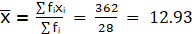Therefore, mean of the given data = 12.93.

4. The following table gives the number of pages written by Sarika for completing her own book for 30 days :

Number of pages written per day 16-18 19-21 22-24 25-27 28-30

Number of days 1 3 4 9 13

Find the mean number of pages written per day.

Solution:

 Class Marks Mid – Value (xi) Number of days (fi) fixi 15.5 – 18.5 17 1 17 18.5 – 21.5 20 3 60 21.5 – 24.5 23 4 92 24.5 – 27.5 26 9 234 27.5 – 30.5 29 13 377 Σfi = 30 Σfixi = 780

The given data is not continuous.

Hence, we subtract 0.5 from the lower limit and add 0.5 in the upper limit of each class.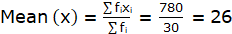Therefore, the mean of pages written per day = 26.

5. The daily income of a sample of 50 employees are tabulated as follows :

Income (in Rs) 1-200 201-400 401-600 601-800

Number of employees 14 15 14 7

Find the mean daily income of employees.

Solution:

 C.I xi di = (xi – a) Fi fidi 1 – 200 100.5 – 200 14 – 2800 201 – 400 300.5 0 15 0 401 – 600 500.5 200 15 2800 601 – 800 700.5 400 7 2800 Σfi = 50 Σfixi = 2800

∴ Assumed mean, a = 300.5 and di = (xi – a)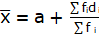= 300.5 + 2800/50

= 356.5

Hence, the average daily income of employees = Rs.356.5

6. An aircraft has 120 passenger seats. The number of seats occupied during 100 flights is given in the following table :

Number of seats 100-104 104-108 108-112 112-116 116-120

Frequency 15 20 32 18 15

Determine the mean number of seats occupied over the flights.

Solution:

 Class Interval Class Marks (xi) Frequency (fi) Deviation (di = xi – a) fidi 100 – 104 102 15 – 8 – 120 104 – 108 106 20 – 4 – 80 108 – 112 110 32 0 0 112 – 116 114 18 4 72 116 – 120 118 15 8 120 N = Σfi = 100 Σfidi = – 8

∴ Assumed mean, a = 110

Class width, h = 4

And total observations, N = 100

Hence, finding mean,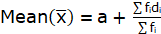= 110 + (-8/100)

= 110 – 0.08

= 109.92

But we know that the seats cannot be in decimal.

Therefore, the number of seats = 109.

7. The weights (in kg) of 50 wrestlers are recorded in the following table :

 Weight (in kg) 100-110 110-120 120-130 130-140 140-150 Number of wrestlers 4 14 21 8 3

Find the mean weight of the wrestlers.

Solution:

 Weight (in kg) Number of Wrestlers (fi) Class Marks (xi) Deviation (di = xi – a) fidi 100 – 110 4 105 – 20 – 80 110 – 120 14 115 – 10 – 140 120 – 130 21 125 0 0 130 – 140 8 135 10 80 140 – 150 3 145 20 60 N = Σfi = 50 Σfidi = – 80

∴ Assumed mean, (a) = 125

Class width, (h) = 10

and total observations, (N) = 50

By step deviation method,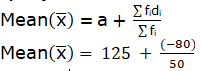= 125 – 16

= 123.4kg

Hence, mean weight of wrestlers = 123.4kg

8. The mileage (km per litre) of 50 cars of the same model was tested by a manufacturer and details are tabulated as given below :

Mileage (km/l) 10-12 12-14 14-16 16-18

Number of cars 7 12 18 13

Find the mean mileage.

The manufacturer claimed that the mileage of the model was 16 km/litre. Do you agree with this claim?

Solution:

 Mileage (km L-1) Class –Marks (xi) Number of cars (fi) fixi 10 – 12 11 7 77 12 – 14 13 12 156 14 – 16 15 18 270 16 – 18 17 13 221 Total Σfi = 50 Σfixi = 724

Here, Σfi = 50

Σfixi = 724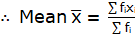= 724/50 = 14.48

Hence, mean mileage = 14.48 km/h

No, I don’t agree with the claim because the manufacturer is claiming mileage 1.52 km/h more than average mileage.

9. The following is the distribution of weights (in kg) of 40 persons :

Weight (in kg) 40-45 45-50 50-55 55-60 60-65 65-70 70-75 75-80

Number of persons 4 4 13 5 6 5 2 1

Construct a cumulative frequency distribution (of the less than type) table for the data above.

Solution:

 Weight (in kg) Cumulative frequency Less than 45 4 Less than 50 4 + 4 = 8 Less than 55 8 + 13 = 21 Less than 60 21 + 5 = 26 Less than 65 26 + 6 = 32 Less than 70 32 + 5 = 37 Less than 75 37 + 2 = 39 Less than 80 39 + 1 = 40

10. The following table shows the cumulative frequency distribution of marks of 800 students in an examination:

Marks Number of students

Below 10 10

Below 20 50

Below 30 130

Below 40 270

Below 50 440

Below 60 570

Below 70 670

Below 80 740

Below 90 780

Below 100 800

Construct a frequency distribution table for the data above.

Solution:

The frequency distribution table for the given data is:

 Class Interval Number of students 0-10 10 10-20 50 – 10 = 40 20-30 130 – 50 = 80 30-40 270 – 130 = 140 40-50 440 – 270 = 170 50-60 570 – 440 = 130 60-70 670 – 570 = 100 70-80 740 – 670 = 70 80-90 780 – 740 = 40 90-100 – 780 = 20

11. Form the frequency distribution table from the following data :

Marks (out of 90) Number of candidates

More than or equal to 80 4

More than or equal to 70 6

More than or equal to 60 11

More than or equal to 50 17

More than or equal to 40 23

More than or equal to 30 27

More than or equal to 20 30

More than or equal to 10 32

More than or equal to 0 34

Solution:

The frequency distribution table for the given data is:

 Class Interval Number of students 0-10 34 – 32 = 2 10-20 32 – 30 = 2 20-30 30 – 27 = 3 30-40 27 – 23 = 4 40-50 23 – 17 = 6 50-60 17 – 11 = 6 60-70 11 – 6 = 5 70-80 6 – 4 = 2 80-90

12. Find the unknown entries a, b, c, d, e, f in the following distribution of heights of students in a class:

Height Frequency Cumulative frequency

(in cm)

150-155 12 a

155-160 b 25

160-165 10 c

165-170 d 43

170-175 e 48

175-180 2 f

Total 50

Solution:

 Height (in cm) Frequency Cumulative frequency given Cumulative frequency 150 – 155 12 a 12 155 – 160 b 25 12 + b 160 – 165 10 c 22 + b 165 – 170 d 43 22 + b + d 170 – 175 e 48 22 + b + d + e 175 – 180 2 f 24 + b + d + e Total 50

On comparing last two tables, we get

a = 12

∴ 12 + b = 25

⇒ b = 25 – 12 = 13

22 + b = c

⇒ c = 22 + 13 = 35

22 + b + d = 43

⇒ 22 + 13 + d = 43

⇒ d = 43 – 35 = 8

22 + b + d + e = 48

⇒ 22 + 13 + 8 + e = 48

⇒ e = 48 – 43 = 5

24 + b + d + e = f

⇒ f = 24 + 13 + 8 + 5 = 50

13. The following are the ages of 300 patients getting medical treatment in a hospital on a particular day:

Age (in years) 10-20 20-30 30-40 40-50 50-60 60-70

Number of patients 60 42 55 70 53 20

Form:

ALess than type cumulative frequency distribution.

• More than type cumulative frequency distribution.Solution:(i)Less than type cumulative frequency distribution of the data is given below.
 (i) Less than type Age (in year) Number of patients Less than 10 0 Less than 20 60 + 0 = 60 Less than 30 60 + 42 = 102 Less than 40 102 + 55 = 157 Less than 50 157 + 70 = 227 Less than 60 227 + 53 = 280 Less than 70 280 + 20 =300

(ii)

More than type cumulative frequency distribution of the data is given below.

 (i) More than type Age (in year) Number of patients More than or equals 10 60 + 42 + 55 + 70 + 53 + 20 = 300 More than or equals 20 42 + 55 + 70 + 53 + 20 = 240 More than or equals 30 55 + 70 + 53 + 20 = 198 More than or equals 40 70 + 53 + 20 = 143 More than or equals 50 53 + 20 = 73 More than or equals 60 20 More than or equals 70 0

14. Given below is a cumulative frequency distribution showing the marks secured by 50 students of a class:

Marks Below 20 Below 40 Below 60 Below 80 Below 100

Number of students 17 22 29 37 50

Form the frequency distribution table for the data.

Solution:

The frequency distribution table for given data.

 Marks Number of students 0 – 20 12 20 – 40 22 – 17 = 5 40 – 60 29 – 22 = 7 60 – 80 37 – 29 = 8 80 – 100 50 – 37 = 13

15. Weekly income of 600 families is tabulated below :

Weekly income Number of families

(in Rs)

0-1000 250

1000-2000 190

2000-3000 100

3000-4000 40

4000-5000 15

5000-6000 5

Total 600

Compute the median income.

Solution:

 Weekly Income Number of families (fi) Cumulative frequency (cf) 0-1000 250 250 1000-2000 190 250 + 190 = 400 2000-3000 100 440 + 100 = 540 3000-4000 40 540 + 40 = 580 4000-5000 15 580 + 15 = 595 5000-6000 5 595 + 5 = 600

According to the question,

n = 600

∴ n/2 = 600/2 = 300

Cumulative frequency 440 lies in the interval 1000 – 2000.

Hence, lower median class, l = 1000

f = 190,

cf = 250,

Class width, h = 1000

And total observation n = 600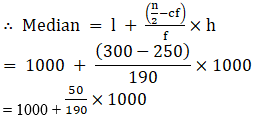= 1000 + 5000/19

= 1000 + 263.15 = 1263.15

Hence, the median income is Rs.1263.15.

16. The maximum bowling speeds, in km per hour, of 33 players at a cricket coaching centre are given as follows:

Speed (km/h) 85-100 100-115 115-130 130-145

Number of players 11 9 8 5

Calculate the median bowling speed.

Solution:

First we construct the cumulative frequency table

 Speed ( in km/h) Number of players Cumulative frequency 85 – 100 11 11 100 – 115 9 11 + 9 = 20 115 – 130 8 20 + 8 = 28 130 – 145 5 28 + 5 = 33

It is given that, n = 33

∴ n/2 = 33/2 = 16.5

Hence, the median class is 100 – 115.

Where, lower limit(l) = 100

Frequency (f) = 9

Cumulative frequency (cf) = 11

And class width(h) = 15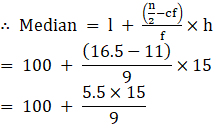= 100 + 82.5/9

= 100 + 9.17

= 109.17

Hence, the median bowling speed is 109.17 km/h.

17. The monthly income of 100 families are given as below :

Income (in Rs) Number of families

0-5000 8

5000-10000 26

10000-15000 41

15000-20000 16

20000-25000 3

25000-30000 3

30000-35000 2

35000-40000 1

Calculate the modal income.

Solution:

According to the data given,

The highest frequency = 41,

41 lies in the interval 10000 – 15000.

Here, l = 10000, fm = 41,f1 = 26,f2 = 16 and h = 5000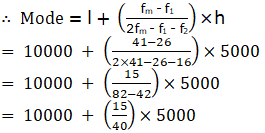= 10000 + 15×125

= 10000 + 1875

= 11875

Hence, the modal income = Rs.11875 per month.

18. The weight of coffee in 70 packets are shown in the following table :

Weight (in g) Number of packets

200-201 12

201-202 26

202-203 20

203-204 9

204-205 2

205-206 1

Determine the modal weight.

Solution:

In the given data, the highest frequency is 26, which lies in the interval 201 – 202

Here, l = 201,fm = 26,f1 = 12,f2 = 20 and (class width) h = 1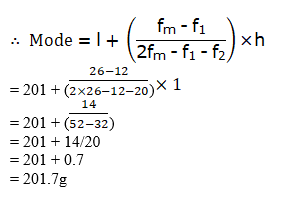Hence, the modal weight = 201.7 g.

19. Two dice are thrown at the same time. Find the probability of getting

ASame number on both dice.
Different numbers on both dice.

Solution:

Two dice are thrown at the same time.

So, total number of possible outcomes = 36

(i) Same number on both dice.

Possible outcomes = (1,1), (2,2), (3, 3), (4, 4), (5, 5), (6, 6).

Hence, number of possible outcomes = 6

Therefore, the probability of getting same number on both dice = 6/36 = 1/6

(ii) Different number on both dice.

Hence, number of possible outcomes

= 36 – Number of possible outcomes for same number on both dice

= 36 – 6 = 30

Therefore, the probability of getting different number on both dice = 30/36 = 5/6

20. Two dice are thrown simultaneously. What is the probability that the sum of the numbers appearing on the dice is

(i) 7? (ii) a prime number? (iii) 1?

Solution:

According to the question,

Two dice are thrown simultaneously.

So, that number of possible outcomes = 36

(i) Sum of the numbers appearing on the dice is 7.

So, the possible outcomes = (1, 6), (2,5), (3, 4), (4, 3), (5, 2), (6, 1).

Hence, number of possible outcomes = 6

∴ the probability that the sum of the numbers appearing on the dice is 7 = 6/36 = 1/6

(ii) Sum of the numbers appearing on the dice is a prime number i.e., 2, 3, 5, 7 and 11.

So, the possible outcomes are (1, 1), (1,2), (2, 1), (1, 4), (2, 3), (3, 2), (4, 1), (1, 6), (2, 5), (3, 4), (4, 3), (5, 2), (6, 1), (5, 6) = (6, 5).

Hence, number of possible outcomes = 15

∴ the probability that the sum of the numbers appearing on the dice is a prime number = 15/36 = 5/12

(iii) Sum of the numbers appearing on the dice is 1.

It is not possible, so its probability is zero.

∴ the probability that the sum of the numbers appearing on the dice is 1 = 0

21. Two dice are thrown together. Find the probability that the product of the numbers on the top of the dice is

(i) 6 (ii) 12 (iii) 7

Solution:

Number of total outcomes = 36

(i) When product of the numbers on the top of the dice = 6.

The possible outcomes = (1, 6), (2,3), (3, 2), (6, 1).

Hence, number of possible ways = 4

∴ Probability that the product of the numbers on the top of the dice is 6= 4/36 = 1/9

(ii) When product of the numbers on the top of the dice = 12.

The possible ways are (2, 6), (3,4), (4, 3), (6, 2).

Hence, number of possible ways = 4

∴ Probability that the product of the numbers on the top of the dice is 12= 4/36 = 1/9

(iii) Product of the numbers on the top of the dice cannot be 7.

Hence, the probability is zero.

∴ Probability that the product of the numbers on the top of the dice is 7 = 0

To facilitate easy learning and help students understand the chapter in full detail, free NCERT Exemplar for Class 10 Maths Chapter 13 is provided here which can be further downloaded in the form of a PDF. Students can also download the other source of learning from BYJU’S such as notes, exemplar books, NCERT Maths Solutions for Class 10 and question papers to prepare for their exams and score good marks. Solving sample papers and previous year question papers also gives an idea of question types asked in the board exam from chapter Chapter 13 Statistics and Probability.

Download BYJU’S – The Learning App and get personalized videos, explaining different types of Maths topics such as statistics, probability, etc. and experience a new way of learning to understand the concepts easily. Also, get Maths Exemplar Solutions for 10th standard, all the chapters here.

## Frequently Asked Questions on NCERT Exemplar Solutions for Class 10 Maths Chapter 13

### List out the topics and sub topics covered in Chapter 13 of NCERT Exemplar Solutions for Class 10 Maths.

The topics and sub topics covered in Chapter 13 of NCERT Exemplar Solutions for Class 10 Maths are listed below.
1. Determining the mean of grouped data by a direct method, assumed mean method, step-deviation method
2. Finding the mode of the given data
3. To find the median of the grouped data
4. Representation of Cumulative Frequency Distribution graphically

### Explain the concept of mean in Chapter 13 of NCERT Exemplar Solutions for Class 10 Maths.

Mean is nothing but the average of the given set of values. It denotes the equal distribution of values for a given data set. Central tendency is the statistical measure that recognizes a single value as representative of the entire distribution. It strives to provide an exact description of the whole data. It is the unique value that represents collected data. The mean, median and mode are the three commonly used measures of central tendency.

### Why is NCERT Exemplar Solutions for Class 10 Maths Chapter 13 beneficial for the students to score well in the board exams?

NCERT Exemplar Solutions for Class 10 Maths Chapter 13 is provided by the experts at BYJU’S based on the understanding abilities of students. The solution module PDF can be downloaded from BYJU’S based on the requirements of students. All the important concepts from the exam point of view are explained in a simple language to help students ace the exam without fear. The solutions for all the problems present in the NCERT textbook helps students to cross check their answers and analyse their areas of weakness.